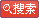# GMATЪ§бЇ--ЫуЪѕИХЪіЃЈШ§ЃЉ

ЗЂВМЪБМфЃК2007-9-3ЁЁЮФзжДѓаЁЃКДѓ жа аЁЁЁДђгЁЃКДђгЁДЫЮФ

АЫ.УшЪіЭГМЦ(descriptive statistics)

1.ЦНОљЪ§(average or arithmetic mean)

2.жаЪ§(median)

To calculate the median of n numbersЃЌfirst order the numbers from least to greatestЃЛif n is oddЃЌthe median is defined as the middle numberЃЌwhile if n is evenЃЌthe median is defined as the average of the two middle numbers. For the data 6, 4, 7, 10, 4, the numbers, in order, are 4, 4, 6, 7, 10, and the median is 6, the middle number. For the numbers 4, 6, 6, 8, 9, 12, the median is (6+8 )/2 = 7. Note that the mean of these numbers is 7.5.

3.жкЪ§(mode)ЃКвЛзщЪ§жаЕФжкЪ§ЪЧжИГіЯжЦЕТЪзюИпЕФЪ§ЁЃ

Р§ЃКthe mode of 7ЃЌ9ЃЌ6ЃЌ7ЃЌ2ЃЌ1 is 7ЁЃ

4.жЕгђ(range)ЃКБэУїЪ§ЕФЗжВМЕФСПЃЌЦфБЛЖЈвхЮЊзюДѓжЕМѕзюаЁжЕЕФВюЁЃ

Р§ЃКthe range ofЈC1ЃЌ7ЃЌ27ЃЌ27ЃЌ36 is 36-(-1)= 37ЁЃ

5.БъзМЗНВю(standard deviation)ЃК

One of the most common measures of dispersion is the standard deviation. Generally speaking, the greater the data are spread away from the mean, the greater the standard deviation. The standard deviation of n numbers can be calculated as follows:

(1)find the arithmetic mean ;

(2)find the differences between the mean and each of the n numbers ;

(3)square each of the differences ;

(4)find the average of the squared differences ;

(5)take the nonnegative square root of this average.

Notice that the standard deviation depends on every data value, although it depends most on values that are farthest from the mean. This is why a distribution with data grouped closely around the mean will have a smaller standard deviation than data spread far from the mean.

6.ХХСагызщКЯ

There are some useful methods for counting objects and sets of objects without actually listing the elements to be counted. The following principle of Multiplication is fundamental to these methods.

If a first object may be chosen in m ways and a second object may be chosen in n ways, then there are mn ways of choosing both objects.

As an example, suppose the objects are items on a menu. If a meal consists of one entree and one dessert and there are 5 entrees and 3 desserts on the menu, then 5ЁС3 = 15 different meals can be ordered from the menu. As another example, each time a coin is flipped, there are two possible outcomes, heads and tails. If an experiment consists of 8 consecutive coin flips, the experiment has 28 possible outcomes, where each of these outcomes is a list of heads and tails in some order.

ЁюНзГЫЃКfactorial notation

МйШчвЛИіДѓгк1ЕФећЪ§nЃЌМЦЫуnЕФНзГЫБЛБэЪОЮЊn!ЃЌБЛЖЈвхЮЊДг1жСnЫљгаећЪ§ЕФГЫЛ§ЃЌ

Р§ШчЃК4! = 4ЁС3ЁС2ЁС1= 24

зЂвтЃК0! = 1! = 1

ЁюХХСаЃКpermutations

The factorial is useful for counting the number of ways that a set of objects can be ordered. If a set of n objects is to be ordered from 1st to nth, there are n choices for the 1st object, n-1 choices for the 2nd object, n-2 choices for the 3rd object, and so on, until there is only 1 choice for the nth object. Thus, by the multiplication principle, the number of ways of ordering the n objects is

n (n-1) (n-2)Ё­( 3) (2) (1) = n!

For example, the number of ways of ordering the letters A, B, and C is 3!, or 6ЃКABC, ACB, BAC, BCA, CAB, and CBA.

These orderings are called the permutations of the letters A, B, and C.вВПЩвдгУP 33БэЪО.

Pkn = n!/ (n-k)!

Р§ШчЃК1, 2, 3, 4, 5ет5ИіЪ§зжЙЙГЩВЛЭЌЕФ5ЮЛЪ§ЕФзмЪ§ЮЊ5! = 120

ЁюзщКЯЃКcombination

A permutation can be thought of as a selection process in which objects are selected one by one in a certain order. If the order of selection is not relevant and only k objects are to be selected from a larger set of n objects, a different counting method is employed.

Specially consider a set of n objects from which a complete selection of k objects is to be made without regard to order, where 0ЁмkЁмn . Then the number of possible complete selections of k objects is called the number of combinations of n objects taken k at a time and is Ckn.

ДгnИідЊЫижаШЮбЁkИідЊЫиЕФЪ§ФПЮЊ:

Ckn. = n!/ (n-k)! k!

Р§ШчЃКДг5ИіВЛЭЌдЊЫижаШЮбЁ2ИіЕФзщКЯЮЊC25 = 5!/2! 3!= 10

ХХСазщКЯЕФвЛаЉЬиад(properties of permutation and combination)

ЁЁ